RRB JE ECE (CBT I) Mock Test- 3

# RRB JE ECE (CBT I) Mock Test- 3

Test Description

## 100 Questions MCQ Test RRB JE Mock Test Series for ECE | RRB JE ECE (CBT I) Mock Test- 3

RRB JE ECE (CBT I) Mock Test- 3 for Railways 2022 is part of RRB JE Mock Test Series for ECE preparation. The RRB JE ECE (CBT I) Mock Test- 3 questions and answers have been prepared according to the Railways exam syllabus.The RRB JE ECE (CBT I) Mock Test- 3 MCQs are made for Railways 2022 Exam. Find important definitions, questions, notes, meanings, examples, exercises, MCQs and online tests for RRB JE ECE (CBT I) Mock Test- 3 below.
Solutions of RRB JE ECE (CBT I) Mock Test- 3 questions in English are available as part of our RRB JE Mock Test Series for ECE for Railways & RRB JE ECE (CBT I) Mock Test- 3 solutions in Hindi for RRB JE Mock Test Series for ECE course. Download more important topics, notes, lectures and mock test series for Railways Exam by signing up for free. Attempt RRB JE ECE (CBT I) Mock Test- 3 | 100 questions in 90 minutes | Mock test for Railways preparation | Free important questions MCQ to study RRB JE Mock Test Series for ECE for Railways Exam | Download free PDF with solutions
 1 Crore+ students have signed up on EduRev. Have you?
RRB JE ECE (CBT I) Mock Test- 3 - Question 1

### Find the next term in the given series?BY, DW, GT, KP,?

Detailed Solution for RRB JE ECE (CBT I) Mock Test- 3 - Question 1 The first letter is increased by 2, 3, 4, 5 and the second letter is decreased by 2, 3, 4, 5. Hence the next term is K+5, P-5 = PK
RRB JE ECE (CBT I) Mock Test- 3 - Question 2

### D and E are the mid-points of AB and AC of ∆ABC, BC is produced to any point P; DE, DP and EP are joined. then, area of:

Detailed Solution for RRB JE ECE (CBT I) Mock Test- 3 - Question 2 (By mid-point theorem)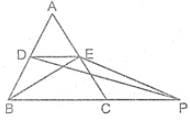DE ∥ BC

DE = 1/2 BC

⇒ ar(∆BDE) = 1/4 × ar(∆ABC)

And ∆BDE = ∆PED

[ ∵ both triangles lie on the same base DE and between two parallel lines DE and BP.]

∴ ar(∆PED) = 1/4 × ar(∆ABC)

RRB JE ECE (CBT I) Mock Test- 3 - Question 3

### One side other than the hypotenuse of the right angle isosceles triangle is 6 cm. The length of the perpendicular on the hypotenuse from the opposite vertex is:

Detailed Solution for RRB JE ECE (CBT I) Mock Test- 3 - Question 3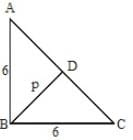Let ∠B be the right angle in ∆ABC

And BD be perpendicular on hypotenuse AC then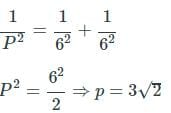RRB JE ECE (CBT I) Mock Test- 3 - Question 4

The speeds of two trains are in the ratio 3: 4. They are going in opposite directions along parallel tracks. If each takes 3 seconds to cross a telegraph post, find the time taken by the trains to cross each other completely?

Detailed Solution for RRB JE ECE (CBT I) Mock Test- 3 - Question 4

Speed of both trains = 3 : 4

x 3 ↓ : ↓ x 3 → time

Length = 9m : 12m.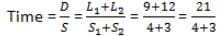= 3 sec.

RRB JE ECE (CBT I) Mock Test- 3 - Question 5

A mother is 3 times faster than her daughter. If the daughter completes a piece of work in 15 days, how long will it take for both mother and daughter to complete the same work?

Detailed Solution for RRB JE ECE (CBT I) Mock Test- 3 - Question 5 Daughter’s one-day work = 1/15

Mother’s one-day work = 1/5

Both can complete their work = 1/5+1/15 = (3+1)/15 = 4/15

Hence, required days= 15/4 days .

RRB JE ECE (CBT I) Mock Test- 3 - Question 6

Study the following table carefully to answer the questions that follow.

The number of soldiers (in thousands) joining five different forces during six different years.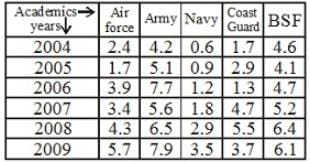The total number of soldiers joining BSF in the years 2004, 2005 and 2006 was approximately what percent of the total number of soldiers joining the Navy over all the years together?

Detailed Solution for RRB JE ECE (CBT I) Mock Test- 3 - Question 6 Total no. of soldiers joining BSF in 2004, 2005, 2006

= 13400

Total no. of soldiers joining in Navy = 10900

Required Percentage= (13400/10900)×100 = 123%

RRB JE ECE (CBT I) Mock Test- 3 - Question 7

Study the following table carefully to answer the questions that follow.

The number of soldiers (in thousands) joining five different forces during six different years.What was the ratio of the number of soldiers joining the Army in the year 2008 to the number of soldiers joining the coast guard in the year 2006?

Detailed Solution for RRB JE ECE (CBT I) Mock Test- 3 - Question 7

Required ratio = 6.5/1.3 = 5/1 = 5:1

RRB JE ECE (CBT I) Mock Test- 3 - Question 8

If tan (5x – 10°) = cot (5y + 20°), then the value of (x+y) is:

Detailed Solution for RRB JE ECE (CBT I) Mock Test- 3 - Question 8 tan (5x – 10°) = cot (5y + 20°)

tan (5x – 10°) = tan (90° – {5y + 20°})

5x – 10° = 90° – (5y + 20°)

5x + 5y = 90° + 10° – 20°

5x + 5y = 80°

x + y = 16°

RRB JE ECE (CBT I) Mock Test- 3 - Question 9

If a train, with a speed of 60 km/hr, crossed a pole in 30 seconds, the length of the train (in meters) is:

Detailed Solution for RRB JE ECE (CBT I) Mock Test- 3 - Question 9 Speed = 60 km/hr

S = 60 x 5/18 m./sec. = 50/3 m./sec.

T = 30 sec.

Length = S x T = (50/3 x 30) m.

/ = 500m.

RRB JE ECE (CBT I) Mock Test- 3 - Question 10

The supplement of an angle is one-fourth of itself. Determine the angle and its supplement.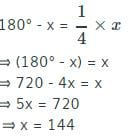Detailed Solution for RRB JE ECE (CBT I) Mock Test- 3 - Question 10 Let the measure of the angle be x°

Then, the measure of its supplementary angle is (180 - x°).

It is given that,

180° - x = 1/4 * x

⟹ (180° - x) = x

⟹ 720 - 4x = x

⟹ 5x = 720

⟹ x = 144

Thus, the measure of the angle is 144° and the measure of its supplement

= 180° - 144° = 36°.

RRB JE ECE (CBT I) Mock Test- 3 - Question 11

3 years ago the average age of a family of 5 members was 17 years. A baby having been born, the average age of the family is the same today. The present age of the baby is

Detailed Solution for RRB JE ECE (CBT I) Mock Test- 3 - Question 11 Total age of 5 members, three years ago

= 17 × 5 = 85 years

Three years hence,

Total age of 5 members = 85+3x5 = 85+15 = 100 years

Sum of present ages of 6 members = 17 × 6 = 102 years

Present age of baby= 102 – 100 = 2 years

RRB JE ECE (CBT I) Mock Test- 3 - Question 12

A ladder leans against a vertical wall. The top of the ladder is 8 metres above the ground. When the bottom of the ladder is moved 2 metres farther away from the wall, the top of the ladder rests against the foot of the wall. What is the length of the ladder?

Detailed Solution for RRB JE ECE (CBT I) Mock Test- 3 - Question 12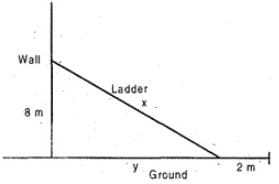Let the length of the ladder be x meter. We have

82 + y2 = x2 and (y + 2) = x

Hence, 64 + (x - 2)2 = x2

= 64 + x2 — 4x + 4 = x2

= 68 = 4x = x = 17 meter

RRB JE ECE (CBT I) Mock Test- 3 - Question 13

The length of a Rectangular plot is decreased by 33.33%. By how much % the breadth of the plot will be increased so that the area remains constant?

Detailed Solution for RRB JE ECE (CBT I) Mock Test- 3 - Question 13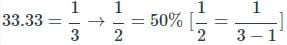RRB JE ECE (CBT I) Mock Test- 3 - Question 14

A man deposited a certain amount in a fixed deposit at r % p.α., interest being compounded annually. If the interest accrued for the fourth and fifth years are Rs 13310 and Rs 14641. what is the total interest accrued for the first three years?

Detailed Solution for RRB JE ECE (CBT I) Mock Test- 3 - Question 14

The interests accrued each year on compound interest form a geometric progression with I, as the first year

interest and common ratio of (1 + r/100)

Therefore, interests for 1st, 2nd, 3rd, 4th and 5th year will be:

I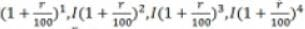respectively.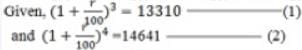(2) divided by (1). we get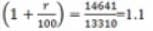∴ Sum of the first three years interests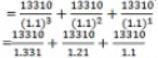= 10000 - 11000 + 12100

= Rs. 33100

RRB JE ECE (CBT I) Mock Test- 3 - Question 15

A person earns Rs. 5000 as an interest in 5/2 years on a certain sum invested with a company at the rate of 10% per annum. Find the sum invested by a person in the company?

Detailed Solution for RRB JE ECE (CBT I) Mock Test- 3 - Question 15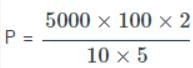P = Rs. 20,000

RRB JE ECE (CBT I) Mock Test- 3 - Question 16

The value of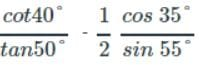is

Detailed Solution for RRB JE ECE (CBT I) Mock Test- 3 - Question 16 cot θ = tan (90 - θ) and cos θ = sin(90 - θ)

Hence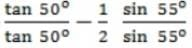1 - 1/2 = 1/2

RRB JE ECE (CBT I) Mock Test- 3 - Question 17

A is twice as fast as B and B is one third as fast as C. If they together can complete work in 30 days. In how many days, A, B and C individually can do the same work?

Detailed Solution for RRB JE ECE (CBT I) Mock Test- 3 - Question 17 Condition 1- A is twice as fast as

B means their ratio is 2: 1.

Condition 2- B is one third as fast

as C, means their ratio is 1: 3. Then we can say, the ratio of A, B and C is as follows

According to condition 1,

A : B = 2 : 1

According to condition 2;

B : C = 1 : 3

Thus, the ratio of A: B: C = 2: 1 : 3

This ratio denotes the working efficiency of A, B and C which means;

A, B and C can do = (2 + 1 + 3 = 6) work/day

Now we can calculate total work i.e.

Total work = Total days × Per day work

Total work = 30 × 6 = 180

A can do the same work in;

= (Total work / Efficiency of A) = 180 / 2 = 90 days

B can do the same work in;

= (Total work / Efficiency of B)

180 / 1 = 180 days

C can do the same work in;

= (Total work / Efficiency of C) = 180 / 3 = 60 days

Thus, A, B and C can individually complete the same work in 90, 180 and 60 days, respectively.

RRB JE ECE (CBT I) Mock Test- 3 - Question 18

If ab = 25, then the minimum value of a + b is:

Detailed Solution for RRB JE ECE (CBT I) Mock Test- 3 - Question 18 Minimum value of (a+ b) can be obtained when a = b

Then a + b = 5 + 5 (∴ ab = 25 and a = b)

= 10

RRB JE ECE (CBT I) Mock Test- 3 - Question 19

If a + b + c = 1, then Find (1+a) (1+b) (1+c)?

Detailed Solution for RRB JE ECE (CBT I) Mock Test- 3 - Question 19 Given, a + b + c = 1

Let observe the value of a, b, c

a = 1/3, b = 1/3, c = 1/3

a + b + c = 1

1/3 + 1/3 + 1/3 = 1

3/3 = 1

1 = 1

put the value of a, b, c

(1 + a) (1 + b) (1 + c)

(1 + 1/3 ) (1 + 1/3 ) (1 + 1/3 )

4/3 x 4/3 x 4/3

64/27 = 2.37

RRB JE ECE (CBT I) Mock Test- 3 - Question 20

The price of a shirt is ₹260 but the shopkeeper successively discounts 15% & 20%. The net sales price is subject to a sales tax of 5%. What does the buyer pay?

Detailed Solution for RRB JE ECE (CBT I) Mock Test- 3 - Question 20

1st discount

S.P = Rs.260 - 15% Rs.260

= 260 - 39 = 221

2nd discount

S.P. = Rs.221 — 20% Rs.221

= 221 - 44.2 = 176.8

Sales tax = 5/100 x 1768/10

= 8840/1000

= Rs.8.84

Amount paid by buyer

= Rs.176.8 + Rs.8.84

= Rs.185.64.

RRB JE ECE (CBT I) Mock Test- 3 - Question 21

A cistern from inside is 12.5 m long, 8.5 m broad and 4 m high and is open at the top. Find the cost of cementing the inside of a cistern at Rs. 24 per sq. m

Detailed Solution for RRB JE ECE (CBT I) Mock Test- 3 - Question 21 Area of surface to be cemented = 2 x (l+b) x h + (lxb)

i.e, area of four walls + area of the floor

= 2 x (21) x 4 + (106.25)

= 274.25 m2

∴ cost of cementing = 24 x 274.25 = Rs. 6582

RRB JE ECE (CBT I) Mock Test- 3 - Question 22

A goat is tied to a pole fixed at a corner outside a room with a square base in a grass field. It is tied using a 14 m long rope. The side of the base of the room is 21 m. Find the area of the field over which the goat can graze (in sq. m).

Detailed Solution for RRB JE ECE (CBT I) Mock Test- 3 - Question 22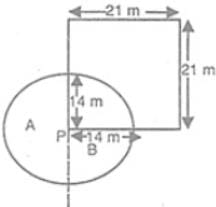Let the goat be tied at P.

The area over which the goat could graze = sum of the areas of the regions A and B = Area of a

The sector of radius 14 m and central angle 270°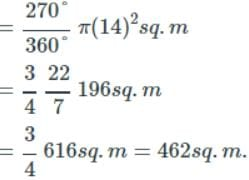RRB JE ECE (CBT I) Mock Test- 3 - Question 23

An article is sold at a loss of 10%. Had it been sold for Rs. 9 more, there would have been a gain of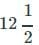% on it. The cost price of the article is :

Detailed Solution for RRB JE ECE (CBT I) Mock Test- 3 - Question 23 Let the cost price of the article = \$ x

S.P. at 10% loss = x × 90/100 = 9x/10

at% gain

S.P. =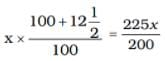According to the question,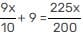⇒ 180x + 1800 = 225x

⇒ 225x – 180x = 1800

⇒ 45x = 1800

⇒ x = \$ 40

We can find required answer with the help of given formula :

Here, a = 10%, R = 9, b = 12.5%

C.P. = R × 100/a + b

C.P. = 12 × 100/12.5 + 10

C.P. = 900/22.5 = \$ 40

RRB JE ECE (CBT I) Mock Test- 3 - Question 24

A water tank is 6 m long, 5 m broad and 3.4 m high. Find the capacity of the tank in litres?

Detailed Solution for RRB JE ECE (CBT I) Mock Test- 3 - Question 24 Volume = 6 x 5 x 3.4

Volume = 102 cu. cm

1 cu. m = 100 cu. cm

102 cu. m = 102 x 100

= 10200 cu. cm

1000 cu. cm = 1 litre

1 cu. cm = 1/1000

10200 cu. cm = (1/1000) x 10200

= 10.2 litres

RRB JE ECE (CBT I) Mock Test- 3 - Question 25

The ratio of the area of a square to that of the square drawn on its diagonal is :

Detailed Solution for RRB JE ECE (CBT I) Mock Test- 3 - Question 25 Let the side of the square be ‘α’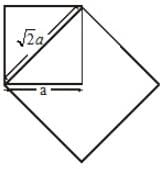Its area = a2

Area of square on the diagonal = (√2a)2 = 2a2

Required ratio = a2/2a2 = 1 : 2

RRB JE ECE (CBT I) Mock Test- 3 - Question 26

If sec θ + tan θ = p, the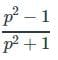= ?

Detailed Solution for RRB JE ECE (CBT I) Mock Test- 3 - Question 26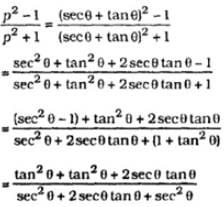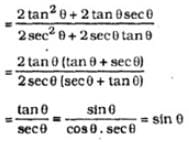RRB JE ECE (CBT I) Mock Test- 3 - Question 27

In the given figure, if ∠ABC = 90° and ∠A = 30°, then ∠ACD=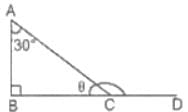Detailed Solution for RRB JE ECE (CBT I) Mock Test- 3 - Question 27

∠ACD = ∠B + ∠A

= 90° + 30°

= 120° (exterior angle)

RRB JE ECE (CBT I) Mock Test- 3 - Question 28

A certain sum of money yields ₹ 1261 as compound interest for 3 years at 5% per annum. The sum is

Detailed Solution for RRB JE ECE (CBT I) Mock Test- 3 - Question 28 Let the principal, compound interest rate and time be x, R and T respectively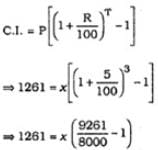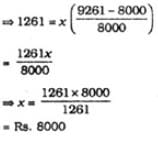RRB JE ECE (CBT I) Mock Test- 3 - Question 29

If 2x-1 + 2x+1 = 320, then x =?

Detailed Solution for RRB JE ECE (CBT I) Mock Test- 3 - Question 29 2x-1 + 2x1 = 320

2x-1 (1 + 22) = 320

2x-1 = 320

2x-1 = 320/5 = 64

2x-1 = 26

x-1 = 6

x = 7

RRB JE ECE (CBT I) Mock Test- 3 - Question 30

The value of (sin2 25° + sin2 65°) is

Detailed Solution for RRB JE ECE (CBT I) Mock Test- 3 - Question 30

sin2 25° + sin2 65° = sin2 (90° – 65°) + sin2 65°

= cos2 65° + sin2 65° = 1.

RRB JE ECE (CBT I) Mock Test- 3 - Question 31

A is twice as good a workman as B. Together, they finish the work in 14 days. In how many days can it be done by each separately?

Detailed Solution for RRB JE ECE (CBT I) Mock Test- 3 - Question 31 As per the question, A does twice the work as done by B.

So A:B = 2:1

Also (A+B)'s one-day work = 1/14

To get days in which B will finish the work,

let's calculate work done by B in 1 day

=(1/14)x(1/3) = 1/42

So B will finish the work in 42 days and A will finish the work in 21 days.

RRB JE ECE (CBT I) Mock Test- 3 - Question 32

There are several chickens and rabbits in a cage (with no other types of animals). There are 72 heads and 200 feet inside the cage. How many chickens and rabbits are there?

Detailed Solution for RRB JE ECE (CBT I) Mock Test- 3 - Question 32 No. of chicken = x

No. of rabbit = y

No. of legs = 2x + 4y = 200

x + 2y = 100 → 1

No. of heads = x + y = 72 → 2

1 - 2

y = 100 - 72 = 28

= 72 - 28 = 44

Hence the correct answer is option A

RRB JE ECE (CBT I) Mock Test- 3 - Question 33

Read the following statements. The conclusions are given in the options, you have to read the conclusions and then decide which one of them among the options definitely does not follow.

Statements:

1. Some tables are chairs.

2. No Chair is a couch.

3. All sofas are couches.

Detailed Solution for RRB JE ECE (CBT I) Mock Test- 3 - Question 33 The Venn diagram of the statements are shown below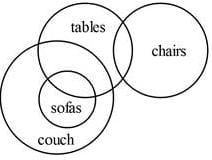All sofas are not chairs. So some sofas being not chairs is true.

So Option 1 is true.

Option 2 is also true

Option 3 is also true.

Option 4 is false.

RRB JE ECE (CBT I) Mock Test- 3 - Question 34

Which of the following will be the mirror image of the given question figure (x), if the mirror is placed along the line MN. Choose the correct mirror image among (I), (II), (III) and (IV) given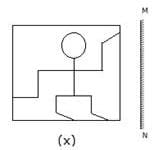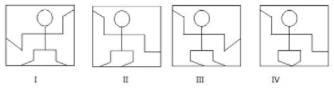Detailed Solution for RRB JE ECE (CBT I) Mock Test- 3 - Question 34 Image II is the correct image of (x).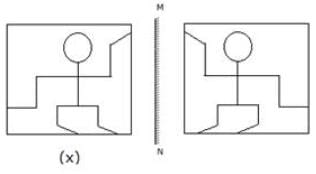RRB JE ECE (CBT I) Mock Test- 3 - Question 35

If in a certain code, GLACIER is coded as 6293017 and FRUIT is coded as 47806, then how will FILTER be coded as in the same code?

Detailed Solution for RRB JE ECE (CBT I) Mock Test- 3 - Question 35 Here, the letters of the word are coded as a certain number. For solving this, we find out the codes for letters from the given codes of the words as follows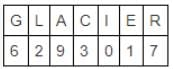And,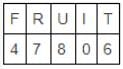So, we get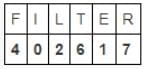RRB JE ECE (CBT I) Mock Test- 3 - Question 36

Harish is standing to the northwest of Kishore. Raju is standing to the southeast of Prem. Prem is standing to the southeast of Kishore. Manu is standing to the east of Kishore. What is the direction of Raju with respect to Harish?

Detailed Solution for RRB JE ECE (CBT I) Mock Test- 3 - Question 36 The positions are shown in the figure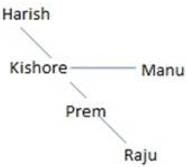Raju South-east of Harish

RRB JE ECE (CBT I) Mock Test- 3 - Question 37

In the following question, select the related numbers from the given alternatives.

9246: 1926 ∷ 3968:?

Detailed Solution for RRB JE ECE (CBT I) Mock Test- 3 - Question 37

9*2 = 18 → 18+1 = 19

4*6 = 24 → 24+2 = 26

Becomes 1926.

Similarly,

3*9 = 27 → 27+1 = 28

6*8 = 48 → 48+2 = 50

Becomes 2850.

RRB JE ECE (CBT I) Mock Test- 3 - Question 38

Arrange the given words in the sequence in which they occur in the dictionary and then choose the correct sequence.

1- Arraign 2- Arrest 3- Arrive 4- Array

Detailed Solution for RRB JE ECE (CBT I) Mock Test- 3 - Question 38 The correct order is arraign, array, arrest, arrive.
RRB JE ECE (CBT I) Mock Test- 3 - Question 39

In order to complete the set, which one of the following set of letters should be placed at the gaps sequentially?

_QOMKI_

Detailed Solution for RRB JE ECE (CBT I) Mock Test- 3 - Question 39 The sequence is letters arranged in reverse alphabetical order, skipping a letter: S, Q, O, M, K, I, G.
RRB JE ECE (CBT I) Mock Test- 3 - Question 40

A Faulty watch loses 5 minutes in 2 hours. It was set right at 6 o’clock in the morning. At 6 o’clock in the morning on the next day, what was the time shown in the faulty watch?

Detailed Solution for RRB JE ECE (CBT I) Mock Test- 3 - Question 40 Time duration = 24 hours

In 2 hours, the faulty watch loses 5 minutes

In 24 hours, the faulty watch loses 60 minutes or 1 hour

The time shown in the faulty watch will be 05:00 a.m.

RRB JE ECE (CBT I) Mock Test- 3 - Question 41

In a certain code, BOTANY is written as Q3M. How is RHYTHM written in that code?

Detailed Solution for RRB JE ECE (CBT I) Mock Test- 3 - Question 41 In this code,

B (2) + O (15) = 17 → Q

T (20) + A (1) = 21 = 2 + 1 = 3

N (14) + Y (25) = 39 = 26 + 13 = M

So, R (18) + H (8) = 26 = Z

Y (25) + T (20) = 45 = 4 + 5 = 9

H (8) + M (13) = 21 → U

Hence, the required code is Z9U

RRB JE ECE (CBT I) Mock Test- 3 - Question 42

Each question given below consists of a statement, followed by two arguments numbered I and II. Decide which of the arguments is a 'strong' argument and which is a 'weak' argument and choose the corresponding option as your answer.

Should the age of drinking be reduced from 21 years to 18 years?

I. Yes, because alcohol is not as harmful to health as smoking is.

II. Yes, because if an 18-year old adult can have the right to vote and get married, he can also have the right to drink

Detailed Solution for RRB JE ECE (CBT I) Mock Test- 3 - Question 42 I is not a strong argument as the statement has not talked about smoking, which is out of scope here. II is a strong argument as both voting and marrying are rights that one gains after turning 18, so being an adult, one must also be able to drink. B is the right answer.
RRB JE ECE (CBT I) Mock Test- 3 - Question 43

Each question given below consists of a statement, followed by two arguments numbered I and II. You have to decide which of the arguments is a 'strong' argument and which is a 'weak' argument.

Statement: Should luxury hotels be banned in India?

Arguments:

I. Yes. They are places from where international criminals operate.

II. No. Affluent foreign tourists will have no place to stay.

Detailed Solution for RRB JE ECE (CBT I) Mock Test- 3 - Question 43 The luxury hotels are a mark of a country's standard and a place for affluent foreign tourists. So, argument II holds. The argument I is not a strong reason because a ban on hotels is not a way to do away with the activities of international criminals.
RRB JE ECE (CBT I) Mock Test- 3 - Question 44

P remembers that his joining date in the office is after 18th October, but not on any odd-numbered date. Q remembers that P is joining in October. R, who is P's friend, remembers that P is joining before 21st October. Then, on which date is P joining?

Detailed Solution for RRB JE ECE (CBT I) Mock Test- 3 - Question 44 P's joining is on an even-numbered date between 18th and 21st, hence, 20th October.
RRB JE ECE (CBT I) Mock Test- 3 - Question 45

Identify the diagram that best represents the relationship among the given classes.

Human, children, Male

(1)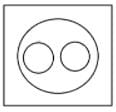(2)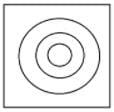(3)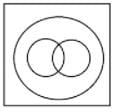(4)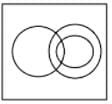Detailed Solution for RRB JE ECE (CBT I) Mock Test- 3 - Question 45

All children are human, and some children are males, all males are humans, thus, 3rd shows the right relationship.

RRB JE ECE (CBT I) Mock Test- 3 - Question 46

In the following question, select the odd letters from the given alternatives.

Detailed Solution for RRB JE ECE (CBT I) Mock Test- 3 - Question 46 The position value of the 2nd alphabet is twice as that of the 1st alphabet. The 3rd alphabet comes just after the 2nd alphabet in the alphabet series

G(7) N(14) O(15)

D(4) H(8) I(9)

I(9) R(18) S(19)

M(13) Y(25) Z(26)

RRB JE ECE (CBT I) Mock Test- 3 - Question 47

Select the related letters/words/numbers from the given alternatives

AK: FP : EO:?

Detailed Solution for RRB JE ECE (CBT I) Mock Test- 3 - Question 47 In this sequence of alphabets, the difference between the numerical value of the 2 alphabets is 10 .i.e.(1 11),(6 16),(5 15), (9 19).
RRB JE ECE (CBT I) Mock Test- 3 - Question 48

Find the odd one out.

Detailed Solution for RRB JE ECE (CBT I) Mock Test- 3 - Question 48

8x4x2 = 64

4x4x4 = 64

2x8x4 = 64

6x2x4 = 64 not 48

RRB JE ECE (CBT I) Mock Test- 3 - Question 49

In the following question, a figure (x) is given having a pattern, whose two portions marked as A and B are missing. Below the figure, four patterns (i), (ii), (iii) and (iv) are given, among which two of them fit A and B correctly. Select the option which matches both A and B correctly.

Question Figure: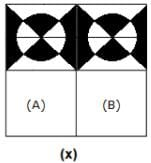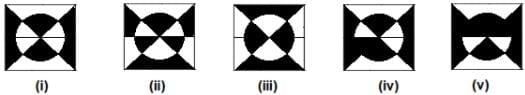Detailed Solution for RRB JE ECE (CBT I) Mock Test- 3 - Question 49 Complete figure is -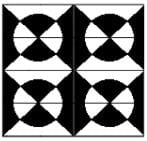RRB JE ECE (CBT I) Mock Test- 3 - Question 50

How many triangles are present in the given figure?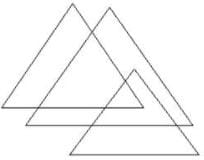Detailed Solution for RRB JE ECE (CBT I) Mock Test- 3 - Question 50 There are 6 triangles in the given figure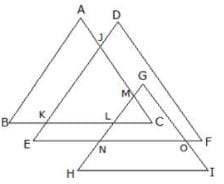Triangles are - ABC, CLM, CKJ, DEF, GHI, GNO.

RRB JE ECE (CBT I) Mock Test- 3 - Question 51

Find out from amongst the four alternatives as to how the pattern would appear on the sheet when the sheet is folded at the dotted line and is punched as given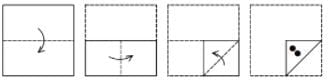Detailed Solution for RRB JE ECE (CBT I) Mock Test- 3 - Question 51 The paper when opened would look like the one given in figure d.
RRB JE ECE (CBT I) Mock Test- 3 - Question 52

Each question given below consists of a statement, followed by two arguments numbered I and II. Decide which of the arguments is a 'strong' argument and which is a 'weak' argument and choose the corresponding option as your answer.

Should sex education be made compulsory at all schools?

I. Yes, because parents are not capable of educating their children.

II. No, because this leads students astray.

Detailed Solution for RRB JE ECE (CBT I) Mock Test- 3 - Question 52 Neither of the two arguments gives valid arguments in favour or against this step, which makes D the right answer.
RRB JE ECE (CBT I) Mock Test- 3 - Question 53

In a queue, Tina is standing 8th from the left and 9th from the right. Pooja is to the immediate left of Sara, who is 8th towards the right of Tina. What is the position of Pooja from the right side?

Detailed Solution for RRB JE ECE (CBT I) Mock Test- 3 - Question 53 Number of students =Position of Tina from left + Position of Tina from right -1=8+9-1=16

Position of Sara = 8+8=16th from left

Position of Pooja = 16-1=15th from left

Position of Pooja from right = 16-15+1=2 from right

RRB JE ECE (CBT I) Mock Test- 3 - Question 54

Three positions of the same cube are shown below. Which number appears on the face opposite to '5'?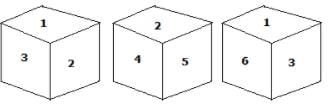Detailed Solution for RRB JE ECE (CBT I) Mock Test- 3 - Question 54 '3' appears opposite to '5'.

We get -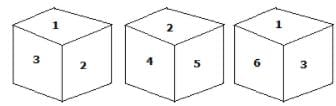RRB JE ECE (CBT I) Mock Test- 3 - Question 55

C is the wife of B and B is the son of K. F is the brother of C and A is the nephew of F. J is the father of F. Q is the daughter of F. How is C related to K?

Detailed Solution for RRB JE ECE (CBT I) Mock Test- 3 - Question 55 C is the wife of B and K is the mother of B. Therefore, C is the daughter in law of K.
RRB JE ECE (CBT I) Mock Test- 3 - Question 56

National Education Day is celebrated in November _____.

Detailed Solution for RRB JE ECE (CBT I) Mock Test- 3 - Question 56 11th November is National Education Day. This day is observed to commemorate the birth anniversary of Maulana Abul Kalam Azad, the first education minister of independent India, who served from 15 August 1947 until 2 February 1958.
RRB JE ECE (CBT I) Mock Test- 3 - Question 57

National Science Day is celebrated every year on:

RRB JE ECE (CBT I) Mock Test- 3 - Question 58

India has been elected as a Member of the ________ for another 4-year term (2019-2022) recently.

Detailed Solution for RRB JE ECE (CBT I) Mock Test- 3 - Question 58 India has been elected as a Member of the International Telecommunications Union (ITU) Council for another 4-year term (2019-2022). The elections to the Council were held during the ongoing ITU Plenipotentiary Conference 2018 at Dubai, UAE.
RRB JE ECE (CBT I) Mock Test- 3 - Question 59

Which of the following is called a ‘Cash Crop’?

RRB JE ECE (CBT I) Mock Test- 3 - Question 60

The third edition of the Indian Ocean Conference was held in -

Detailed Solution for RRB JE ECE (CBT I) Mock Test- 3 - Question 60 The third edition of the Indian Ocean Conference was held in Hanoi, the capital city of Vietnam from 27-28 August 2018.
RRB JE ECE (CBT I) Mock Test- 3 - Question 61

What was the official language of the Sultanate?

RRB JE ECE (CBT I) Mock Test- 3 - Question 62

Which of the following crops are suitable for growing on black soil?

Detailed Solution for RRB JE ECE (CBT I) Mock Test- 3 - Question 62 All of these crops can be grown on black soil
RRB JE ECE (CBT I) Mock Test- 3 - Question 63

The sultan who described himself as “The second Alexander”(Sikander-i-e-Sani’ ) was?

Detailed Solution for RRB JE ECE (CBT I) Mock Test- 3 - Question 63 Alauddin Khilji heard of becoming second Alexander and ordered to engrave his name as second Alexander in his coins. He assumed the title of

"Sikandar -i- Sani"

RRB JE ECE (CBT I) Mock Test- 3 - Question 64

Jharia of Jharkhand is well-known for its -

RRB JE ECE (CBT I) Mock Test- 3 - Question 65

Sher Shah defeated Humayun and captured Gaur in the battle of:

Detailed Solution for RRB JE ECE (CBT I) Mock Test- 3 - Question 65 Sher Shah defeated Humayun in the Battle of Chausa in 1539 CE and captured Gaur.
RRB JE ECE (CBT I) Mock Test- 3 - Question 66

Which one is NOT situated at Fatehpur Sikri?

RRB JE ECE (CBT I) Mock Test- 3 - Question 67

Tropic of Cancer does not pass through -

RRB JE ECE (CBT I) Mock Test- 3 - Question 68

How many National Parties are there in India(as of Jan 2019)?

RRB JE ECE (CBT I) Mock Test- 3 - Question 69

Which place is famous for diamond mining?

RRB JE ECE (CBT I) Mock Test- 3 - Question 70

The longest-serving Governor of the RBI is-

Detailed Solution for RRB JE ECE (CBT I) Mock Test- 3 - Question 70 Holding the office for over seven years, Benegal Rama Rau is the longest-serving governor, while Amitav Ghosh's 20-day term is the shortest.
RRB JE ECE (CBT I) Mock Test- 3 - Question 71

The addition of a neutron to the nucleus of an atom-

Detailed Solution for RRB JE ECE (CBT I) Mock Test- 3 - Question 71 The addition of a neutron to the nucleus of an atom, will increase the atomic mass of the atom.
RRB JE ECE (CBT I) Mock Test- 3 - Question 72

Which catalyst is used in the conversion of sucrose into glucose and fructose?

Detailed Solution for RRB JE ECE (CBT I) Mock Test- 3 - Question 72 Invertase is an enzyme that catalyzes the hydrolysis of sucrose into fructose and glucose. The resulting mixture of fructose and glucose is called inverted sugar syrup.
RRB JE ECE (CBT I) Mock Test- 3 - Question 73

Which of the following belong to the phylum Coelenterata?

Detailed Solution for RRB JE ECE (CBT I) Mock Test- 3 - Question 73 Animals belonging to Coelenterata have thread-like structures called tentacles and hydra, jellyfish and sea anemone belong to this.
RRB JE ECE (CBT I) Mock Test- 3 - Question 74

What happens If an egg with a shell is placed in a microwave oven?

Detailed Solution for RRB JE ECE (CBT I) Mock Test- 3 - Question 74 If an egg with a shell is placed in a microwave oven, the eggshell will explode. It is because there is no control on the rise of temperature while heating in the microwave and the water content in albumen (actually yolk also contains water but it is mainly fat. Albumen has relatively more water content) gets converted into steam and the pressure inside the shell increases. Hence it explodes
RRB JE ECE (CBT I) Mock Test- 3 - Question 75

When a Red Glass Plate and a Blue Glass plate combined, it will transmit ______?

RRB JE ECE (CBT I) Mock Test- 3 - Question 76

Which of the following is not a colloid?

Detailed Solution for RRB JE ECE (CBT I) Mock Test- 3 - Question 76 Chlorophyll is a green pigment essential for photosynthesis. And it is not a colloid
RRB JE ECE (CBT I) Mock Test- 3 - Question 77

If a bus starts from rest then the passenger will ….?

Detailed Solution for RRB JE ECE (CBT I) Mock Test- 3 - Question 77 Due to the inertia of rest, passengers sitting on a motionless bus will move backwards as the bus starts.
RRB JE ECE (CBT I) Mock Test- 3 - Question 78

Lucas Test is associated with….?

Detailed Solution for RRB JE ECE (CBT I) Mock Test- 3 - Question 78 Lucas test is performed to the difference among primary, secondary and tertiary alcohol.
RRB JE ECE (CBT I) Mock Test- 3 - Question 79

Which of the following enzymes is not secreted in the small intestine?

Detailed Solution for RRB JE ECE (CBT I) Mock Test- 3 - Question 79 Pepsin is secreted in the stomach which converts protein into peptones.
RRB JE ECE (CBT I) Mock Test- 3 - Question 80

The SI unit of power—

Detailed Solution for RRB JE ECE (CBT I) Mock Test- 3 - Question 80 The SI unit of power is the watt (W), which is equal to one joule per second.
RRB JE ECE (CBT I) Mock Test- 3 - Question 81

The nitride ion will have-

Detailed Solution for RRB JE ECE (CBT I) Mock Test- 3 - Question 81 A nitride ion has 7 protons, 8 neutrons, and 10 electrons.
RRB JE ECE (CBT I) Mock Test- 3 - Question 82

The unit of Young’s Modulus of Elasticity in the MKS system is?

Detailed Solution for RRB JE ECE (CBT I) Mock Test- 3 - Question 82 Young's modulus is the ratio of stress (which has units of pressure) to strain (which is dimensionless), and so Young's modulus has units of pressure. Its SI unit is therefore the pascal (Pa or N/m2 or kg).
RRB JE ECE (CBT I) Mock Test- 3 - Question 83

What is an electrical fuse used to ________?

Detailed Solution for RRB JE ECE (CBT I) Mock Test- 3 - Question 83 In electronics and electrical engineering, a fuse is an electrical safety device that operates to provide overcurrent protection of an electrical circuit.
RRB JE ECE (CBT I) Mock Test- 3 - Question 84

The life span of RBC is:

Detailed Solution for RRB JE ECE (CBT I) Mock Test- 3 - Question 84 Human red blood cells are formed mainly in the bone marrow and have an average lifespan of approximately 120 days.
RRB JE ECE (CBT I) Mock Test- 3 - Question 85

Choose the one which is different or odd from the following.

Detailed Solution for RRB JE ECE (CBT I) Mock Test- 3 - Question 85 Except aluminium, iron and copper, Brass is an alloy of copper and zinc.
RRB JE ECE (CBT I) Mock Test- 3 - Question 86

A person bakes a cake. It turns out to be hard and small in size. Which ingredient has he forgotten to add that would have caused the cake to rise and become light?

Detailed Solution for RRB JE ECE (CBT I) Mock Test- 3 - Question 86 Baking powder is a dry chemical leavening agent, a mixture of a carbonate or bicarbonate and a weak acid and is used for increasing the volume and lightening the texture of baked goods.
RRB JE ECE (CBT I) Mock Test- 3 - Question 87

Why are clear nights colder than cloudy nights?

Detailed Solution for RRB JE ECE (CBT I) Mock Test- 3 - Question 87

Clouds are made of tiny droplets of liquid or frozen water. Clouds act like blankets that prevent heat energy stored in the air and soil from leaving the Earth in the form of infrared radiation.

RRB JE ECE (CBT I) Mock Test- 3 - Question 88

Melting point of cast iron (inC) is in the range of

Detailed Solution for RRB JE ECE (CBT I) Mock Test- 3 - Question 88 Cast Iron melting point range 1150-1300C
RRB JE ECE (CBT I) Mock Test- 3 - Question 89

Candela is the unit of-

Detailed Solution for RRB JE ECE (CBT I) Mock Test- 3 - Question 89 The luminous intensity, in a given direction, of a source that emits monochromatic radiation of frequency 540×1012 hertz and that has a radiant intensity in that direction of 1/683 watts per steradian.
RRB JE ECE (CBT I) Mock Test- 3 - Question 90

Which acid is used to remove stains?

Detailed Solution for RRB JE ECE (CBT I) Mock Test- 3 - Question 90 Oxalic Acid is used to remove the stain and it is mainly the constituent of tomato.
RRB JE ECE (CBT I) Mock Test- 3 - Question 91

Where shall the water boil at the lowest temperature?

Detailed Solution for RRB JE ECE (CBT I) Mock Test- 3 - Question 91 Water to get a boil, its vapour pressure must reach its surrounding pressure. In a pressure cooker, it boils at the highest temperature. The elevation of Kochi is 0 m, Ooty is 2,486 m, Mount Abu is 1,200 m, New Delhi is 216 m, and Shimla is 2,205 m. So comparing all of them, water will boil at the lowest temperature at Ooty, followed by Shimla, Mount Abu, New Delhi and Kochi. This is because the higher we go, the lower is the atmospheric pressure and the lower is the point where water is vapour pressure gets equal to the atmospheric pressure.
RRB JE ECE (CBT I) Mock Test- 3 - Question 92

The Chemical formula of Caustic Soda is-

Detailed Solution for RRB JE ECE (CBT I) Mock Test- 3 - Question 92 Sodium hydroxide, also known as lye and caustic soda, is an inorganic compound with the formula NaOH.
RRB JE ECE (CBT I) Mock Test- 3 - Question 93

Which of the following types of rays does NOT penetrate Earth's atmosphere?

Detailed Solution for RRB JE ECE (CBT I) Mock Test- 3 - Question 93 X-Rays are not able to penetrate the earth’s thick atmosphere. Hence to image X-rays from celestial bodies, we are sending X-ray telescopes to outer space.
RRB JE ECE (CBT I) Mock Test- 3 - Question 94

Quartz is a metamorphic rock. What is the chemical name of quartz?

Detailed Solution for RRB JE ECE (CBT I) Mock Test- 3 - Question 94 Quartz is a metamorphic form of sandstone which is chemically known as silicon dioxide.
RRB JE ECE (CBT I) Mock Test- 3 - Question 95

Farad is associated with….?

Detailed Solution for RRB JE ECE (CBT I) Mock Test- 3 - Question 95 Farad is the unit of capacitance. 1 Farad is the capacitance if a charge of 1C increases potential difference by 1 volt
RRB JE ECE (CBT I) Mock Test- 3 - Question 96

The resistance of a conductor is inversely proportional to:

Detailed Solution for RRB JE ECE (CBT I) Mock Test- 3 - Question 96 The resistance of a wire is proportional to its length and inversely proportional to its cross-sectional area. The constant of proportionality is called the resistivity of the material making up the wire.
RRB JE ECE (CBT I) Mock Test- 3 - Question 97

Graphite and heavy water are two common moderators used in a nuclear reactor. The function of the moderator is to:

Detailed Solution for RRB JE ECE (CBT I) Mock Test- 3 - Question 97 Heavy water is basically used as a moderator in nuclear reactors to slow down the neutrons so that they are captured and become effective to bring about the fission reaction. The main reason why heavy water is used as a moderator is that it captures less neutrons than normal water.
RRB JE ECE (CBT I) Mock Test- 3 - Question 98

Which of the following is the parasitic plant?

Detailed Solution for RRB JE ECE (CBT I) Mock Test- 3 - Question 98 A parasitic plant is a plant that derives some or all of its nutritional requirements from another living plant.
RRB JE ECE (CBT I) Mock Test- 3 - Question 99

Which of the following substances is also called brain alcohol?

Detailed Solution for RRB JE ECE (CBT I) Mock Test- 3 - Question 99 Ethyl Alcohol is an intoxicant that is formed by the anaerobic respiration of yeast and is also called brain alcohol
RRB JE ECE (CBT I) Mock Test- 3 - Question 100

Which mosquito is responsible for Japanese encephalitis?

Detailed Solution for RRB JE ECE (CBT I) Mock Test- 3 - Question 100 Culex mosquito is active in tropical and subtropical regions and is responsible for Japanese encephalitis.

## RRB JE Mock Test Series for ECE

10 tests
 Use Code STAYHOME200 and get INR 200 additional OFF Use Coupon Code
Information about RRB JE ECE (CBT I) Mock Test- 3 Page
In this test you can find the Exam questions for RRB JE ECE (CBT I) Mock Test- 3 solved & explained in the simplest way possible. Besides giving Questions and answers for RRB JE ECE (CBT I) Mock Test- 3, EduRev gives you an ample number of Online tests for practice

10 tests